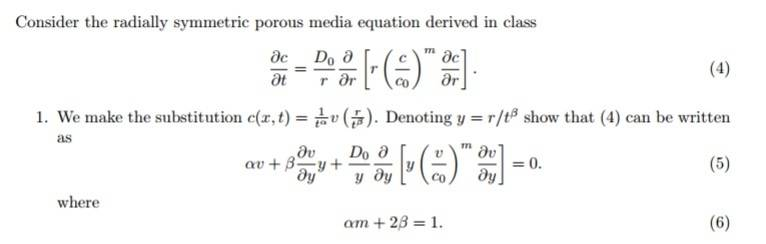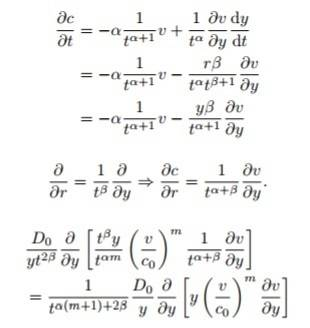# Differential Equation, Change of variables

## Homework Statement

Hi,

I am looking at this question:With this (part of ) solution:## The Attempt at a Solution

I follow up to the last line-

I do not understand here how we have simply taken the ##1/t^{\alpha m + \alpha}## outside of the derivative ##\frac{\partial}{\partial y} ## since ##y=y(r,t^{\beta}) ## i.e. ##t## and ##y## are not independent variables.... ##\frac{\partial}{\partial t}= \frac{\partial y}{\partial t^{\beta}}\frac{\partial t^{\beta}}{\partial t}##

Many thanks in advance

## Answers and Replies

haruspex
Science Advisor
Homework Helper
Gold Member
2020 Award
My problems with the algebra start before that.
A partial derivative expresses the consequences of changing one variable while one or more other variables are held constant. The full notation specifies what is held constant. E.g. if f=f(x,y) then we may write ##\frac {\partial f}{\partial x}\Big\rvert_{y=y_0}##. Nearly always, it is obvious what the 'other' variables are, and we don't need to specify their values, so we omit the vertical bar and its subscript.
In the present case, I have no idea what is being held constant as y varies.